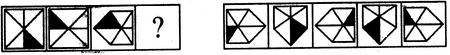Courses

# Analogy Quiz II, Non Verbal Reasoning

## 20 Questions MCQ Test General Aptitude for GATE | Analogy Quiz II, Non Verbal Reasoning

Description
This mock test of Analogy Quiz II, Non Verbal Reasoning for LR helps you for every LR entrance exam. This contains 20 Multiple Choice Questions for LR Analogy Quiz II, Non Verbal Reasoning (mcq) to study with solutions a complete question bank. The solved questions answers in this Analogy Quiz II, Non Verbal Reasoning quiz give you a good mix of easy questions and tough questions. LR students definitely take this Analogy Quiz II, Non Verbal Reasoning exercise for a better result in the exam. You can find other Analogy Quiz II, Non Verbal Reasoning extra questions, long questions & short questions for LR on EduRev as well by searching above.
QUESTION: 1

### Directions to Solve Each of the following questions consists of two sets of figures. Figures A, B, C and D constitute the Problem Set while figures 1, 2, 3, 4 and 5 constitute the Answer Set. There is a definite relationship between figures A and B. Establish a similar relationship between figures C and D by selecting a suitable figure from the Answer Set that would replace the question mark (?) in fig. (D). Question - Select a suitable figure from the Answer Figures that would replace the question mark (?).Problem Figures:                            Answer Figures: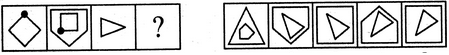(A)     (B)      (C)     (D)                  (1)      (2)      (3)      (4)      (5)

Solution: The figure rotates through 135oACW and is placed inside another figure with one more number of sides.
QUESTION: 2

### Directions to Solve Each of the following questions consists of two sets of figures. Figures A, B, C and D constitute the Problem Set while figures 1, 2, 3, 4 and 5 constitute the Answer Set. There is a definite relationship between figures A and B. Establish a similar relationship between figures C and D by selecting a suitable figure from the Answer Set that would replace the question mark (?) in fig. (D). Question - Select a suitable figure from the Answer Figures that would replace the question mark (?).Problem Figures:                            Answer Figures: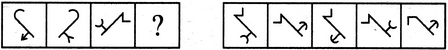(A)     (B)      (C)     (D)                  (1)      (2)      (3)      (4)      (5)

Solution: The figure gets laterally inverted and the head of the arrow (the arrowhead may be a 'V' or an arc) gets inverted.
QUESTION: 3

### Directions to Solve Each of the following questions consists of two sets of figures. Figures A, B, C and D constitute the Problem Set while figures 1, 2, 3, 4 and 5 constitute the Answer Set. There is a definite relationship between figures A and B. Establish a similar relationship between figures C and D by selecting a suitable figure from the Answer Set that would replace the question mark (?) in fig. (D). Question - Select a suitable figure from the Answer Figures that would replace the question mark (?).Problem Figures:                            Answer Figures: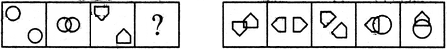(A)     (B)      (C)     (D)                  (1)      (2)      (3)      (4)      (5)

Solution: The two elements approach each other and get overlapped;
QUESTION: 4

Each of the following questions consists of two sets of figures. Figures A, B, C and D constitute the Problem Set while figures 1, 2, 3, 4 and 5 constitute the Answer Set. There is a definite relationship between figures A and B. Establish a similar relationship between figures C and D by selecting a suitable figure from the Answer Set that would replace the question mark (?) in fig. (D).

Question -

Select a suitable figure from the Answer Figures that would replace the question mark (?).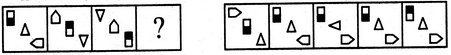(A)     (B)      (C)     (D)                  (1)      (2)      (3)      (4)      (5)
Solution: The elements move downwards along the diagonal and the lowermost element moves to the uppermost position. The triangle and the half shaded rectangle get vertically inverted and the pentagon rotates 90oCW.
QUESTION: 5

Each of the following questions consists of two sets of figures. Figures A, B, C and D constitute the Problem Set while figures 1, 2, 3, 4 and 5 constitute the Answer Set. There is a definite relationship between figures A and B. Establish a similar relationship between figures C and D by selecting a suitable figure from the Answer Set that would replace the question mark (?) in fig. (D).

Question -

Select a suitable figure from the Answer Figures that would replace the question mark (?).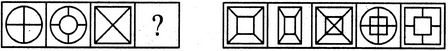(A)     (B)      (C)     (D)                  (1)      (2)      (3)      (4)      (5)
Solution: An element similar to but smaller than the outer element appears as the inner element and it hides the parts of the line segments that come under it.
QUESTION: 6

Each of the following questions consists of two sets of figures. Figures A, B, C and D constitute the Problem Set while figures 1, 2, 3, 4 and 5 constitute the Answer Set. There is a definite relationship between figures A and B. Establish a similar relationship between figures C and D by selecting a suitable figure from the Answer Set that would replace the question mark (?) in fig. (D).

Question -

Select a suitable figure from the Answer Figures that would replace the question mark (?).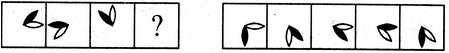(A)     (B)      (C)     (D)                  (1)      (2)      (3)      (4)      (5)
Solution: The black leaf rotates 135oACW and the white leaf rotates 135oCW.
QUESTION: 7

Each of the following questions consists of two sets of figures. Figures A, B, C and D constitute the Problem Set while figures 1, 2, 3, 4 and 5 constitute the Answer Set. There is a definite relationship between figures A and B. Establish a similar relationship between figures C and D by selecting a suitable figure from the Answer Set that would replace the question mark (?) in fig. (D).

Question -

Select a suitable figure from the Answer Figures that would replace the question mark (?).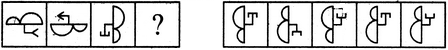(A)     (B)      (C)     (D)                  (1)      (2)      (3)      (4)      (5)
Solution: The figure rotates through 180o and the head of the arrow attached to the main figure, gets inverted.
QUESTION: 8

Each of the following questions consists of two sets of figures. Figures A, B, C and D constitute the Problem Set while figures 1, 2, 3, 4 and 5 constitute the Answer Set. There is a definite relationship between figures A and B. Establish a similar relationship between figures C and D by selecting a suitable figure from the Answer Set that would replace the question mark (?) in fig. (D).

Question -

Select a suitable figure from the Answer Figures that would replace the question mark (?).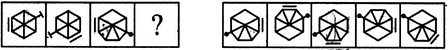(A)     (B)      (C)     (D)                  (1)      (2)      (3)      (4)      (5)
Solution: The line segment along one of the sides of the figure moves two spaces ACW (each space is equal to a side of the figure) and the other element moves three spaces CW.
QUESTION: 9

Each of the following questions consists of two sets of figures. Figures A, B, C and D constitute the Problem Set while figures 1, 2, 3, 4 and 5 constitute the Answer Set. There is a definite relationship between figures A and B. Establish a similar relationship between figures C and D by selecting a suitable figure from the Answer Set that would replace the question mark (?) in fig. (D).

Question -

Select a suitable figure from the Answer Figures that would replace the question mark (?).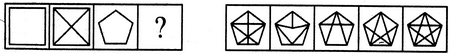(A)     (B)      (C)     (D)                  (1)      (2)      (3)      (4)      (5)
Solution: All the diagonals of the figure have been drawn.
QUESTION: 10

Each of the following questions consists of two sets of figures. Figures A, B, C and D constitute the Problem Set while figures 1, 2, 3, 4 and 5 constitute the Answer Set. There is a definite relationship between figures A and B. Establish a similar relationship between figures C and D by selecting a suitable figure from the Answer Set that would replace the question mark (?) in fig. (D).

Question -

Select a suitable figure from the Answer Figures that would replace the question mark (?).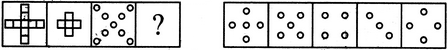(A)     (B)      (C)     (D)                  (1)      (2)      (3)      (4)      (5)
Solution: The four parts at the outer ends of the figure are lost.
QUESTION: 11

Each of the following questions consists of two sets of figures. Figures A, B, C and D constitute the Problem Set while figures 1, 2, 3, 4 and 5 constitute the Answer Set. There is a definite relationship between figures A and B. Establish a similar relationship between figures C and D by selecting a suitable figure from the Answer Set that would replace the question mark (?) in fig. (D).

Question -

Select a suitable figure from the Answer Figures that would replace the question mark (?).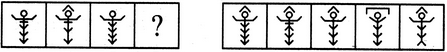(A)     (B)      (C)     (D)                  (1)      (2)      (3)      (4)      (5)
Solution: One of the arrowheads moves from the lower part to the upper part of the figure above the circle and gets vertically inverted.
QUESTION: 12

Each of the following questions consists of two sets of figures. Figures A, B, C and D constitute the Problem Set while figures 1, 2, 3, 4 and 5 constitute the Answer Set. There is a definite relationship between figures A and B. Establish a similar relationship between figures C and D by selecting a suitable figure from the Answer Set that would replace the question mark (?) in fig. (D).

Question -

Select a suitable figure from the Answer Figures that would replace the question mark (?).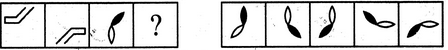(A)     (B)      (C)     (D)                  (1)      (2)      (3)      (4)      (5)
Solution: The figure rotates through 180o.
QUESTION: 13

Each of the following questions consists of two sets of figures. Figures A, B, C and D constitute the Problem Set while figures 1, 2, 3, 4 and 5 constitute the Answer Set. There is a definite relationship between figures A and B. Establish a similar relationship between figures C and D by selecting a suitable figure from the Answer Set that would replace the question mark (?) in fig. (D).

Question -

Select a suitable figure from the Answer Figures that would replace the question mark (?).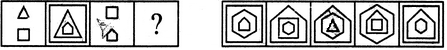(A)     (B)      (C)     (D)                  (1)      (2)      (3)      (4)      (5)
Solution: The lower element gets enlarged and becomes the outermost element; the upper element gets slightly enlarged and becomes the middle element and a new element with one more number of sides than the lower element appears as the innermost element.
QUESTION: 14

Each of the following questions consists of two sets of figures. Figures A, B, C and D constitute the Problem Set while figures 1, 2, 3, 4 and 5 constitute the Answer Set. There is a definite relationship between figures A and B. Establish a similar relationship between figures C and D by selecting a suitable figure from the Answer Set that would replace the question mark (?) in fig. (D).

Question -

Select a suitable figure from the Answer Figures that would replace the question mark (?).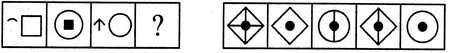(A)     (B)      (C)     (D)                  (1)      (2)      (3)      (4)      (5)
Solution: The LHS element becomes a closed figure by combining with three similar elements. The RHS element gets reduced in size, turns black and moves to the centre of the other figure.
QUESTION: 15

Directions to Solve

Each of the following questions consists of two sets of figures. Figures A, B, C and D constitute the Problem Set while figures 1, 2, 3, 4 and 5 constitute the Answer Set. There is a definite relationship between figures A and B. Establish a similar relationship between figures C and D by selecting a suitable figure from the Answer Set that would replace the question mark (?) in fig. (D).

Question -

Select a suitable figure from the Answer Figures that would replace the question mark (?).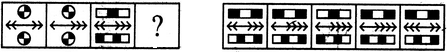(A)     (B)      (C)     (D)                       (1)       (2)        (3)        (4)        (5)

Solution:

In the upper and the lower elements, the white part turns black and the black turns white. In the central arrow, one of the arrowheads from the LHS gets laterally inverted and moves to the RHS.

QUESTION: 16

Each of the following questions consists of two sets of figures. Figures A, B, C and D constitute the Problem Set while figures 1, 2, 3, 4 and 5 constitute the Answer Set. There is a definite relationship between figures A and B. Establish a similar relationship between figures C and D by selecting a suitable figure from the Answer Set that would replace the question mark (?) in fig. (D).

Question -

Select a suitable figure from the Answer Figures that would replace the question mark (?).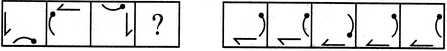(A)     (B)      (C)     (D)                  (1)      (2)      (3)      (4)      (5)
Solution: The half-arrow rotates 90oACW and gets laterally inverted and moves to the adjacent side of the square boundary in a CW direction. The bent pin rotates 90oACW and moves to the adjacent side of the square boundary in a CW direction.
QUESTION: 17

Each of the following questions consists of two sets of figures. Figures A, B, C and D constitute the Problem Set while figures 1, 2, 3, 4 and 5 constitute the Answer Set. There is a definite relationship between figures A and B. Establish a similar relationship between figures C and D by selecting a suitable figure from the Answer Set that would replace the question mark (?) in fig. (D).

Question -

Select a suitable figure from the Answer Figures that would replace the question mark (?).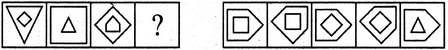(A)     (B)      (C)     (D)                  (1)      (2)      (3)      (4)      (5)
Solution: The inner element gets enlarged, rotates 45oCW and becomes the outer element. The outer element reduces in size, gets vertically inverted and becomes the inner element.
QUESTION: 18

Each of the following questions consists of two sets of figures. Figures A, B, C and D constitute the Problem Set while figures 1, 2, 3, 4 and 5 constitute the Answer Set. There is a definite relationship between figures A and B. Establish a similar relationship between figures C and D by selecting a suitable figure from the Answer Set that would replace the question mark (?) in fig. (D).

Question -

Select a suitable figure from the Answer Figures that would replace the question mark (?).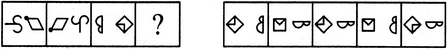(A)     (B)      (C)     (D)                  (1)      (2)      (3)      (4)      (5)
Solution: The two elements interchange positions; the element that reaches the LHS position gets vertically inverted and the element that reaches the RHS position, rotates 90oACW.
QUESTION: 19

Each of the following questions consists of two sets of figures. Figures A, B, C and D constitute the Problem Set while figures 1, 2, 3, 4 and 5 constitute the Answer Set. There is a definite relationship between figures A and B. Establish a similar relationship between figures C and D by selecting a suitable figure from the Answer Set that would replace the question mark (?) in fig. (D).

Question -

Select a suitable figure from the Answer Figures that would replace the question mark (?).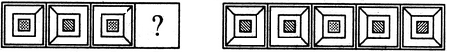(A)     (B)      (C)     (D)                  (1)      (2)      (3)      (4)      (5)
Solution: The existing line segments that join the corners of the squares move to the adjacent corners in an ACW direction and one more line segment appears in the next corner at the ACW-end. The shading inside the innermost square changes from dots to slanting lines.
QUESTION: 20

Each of the following questions consists of two sets of figures. Figures A, B, C and D constitute the Problem Set while figures 1, 2, 3, 4 and 5 constitute the Answer Set. There is a definite relationship between figures A and B. Establish a similar relationship between figures C and D by selecting a suitable figure from the Answer Set that would replace the question mark (?) in fig. (D).

Question -

Select a suitable figure from the Answer Figures that would replace the question mark (?).